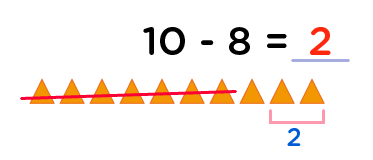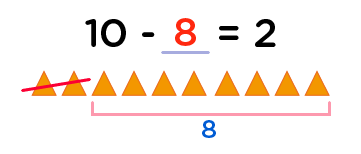Subtraction Within 10 Worksheets for Kindergarten - Free Printable Subtraction Math for Kindergarten

Subtraction To 10 Kindergarten Worksheets

Subtraction within 10 worksheets for kindergarten is the best math resource in which kinders can use to boast an understanding of subtracting numbers up to 10. Moreover, kids will hugely perfect their subtraction skills, and equally enhance their number sense skills.

This is because our free printable subtraction math for kindergarten worksheets have made use of colorful objects to represent the minuend in the subtraction sentence.

As a result, these objects will excite kids, so that they can easily see count, cross out the subtrahend, then count the remaining objects to eventually find out the difference.

This however ties with the fact that kids obtain strong number sense skills when they are able to count and represent quantities with numbers.

Important facts about subtraction up to 10 for kinders

Subtraction to 10 kindergarten worksheets is of vital importance, as it helps kids to easily master and use subtraction skills as they engage in societal issues right from an early age till infinity.

From among countless daily experiences the knowledge of subtraction will enable kids to know the number of marbles his friends took after having counted the ones available.

So, as parents obtain our free printable subtraction math for kindergarten, it will help prevent kid’s frustration of thinking that he has given out almost all his play objects or biscuits to his friends. This skill in kids will continue to be of great help to them in later years as they engage in monetary transactions of balancing, either when buying or selling.

Best strategies to obtain missing parts - subtraction sentences to 10 kindergarten worksheets

As an important technique used in our Subtraction to 10 kindergarten worksheets, kids are required to figure out the missing part in a subtraction sentence. We are probably going to find here best strategies to obtain missing parts.

This idea is in fact an excellent approach, though challenging, but it’s going to strengthen kids understanding of subtraction in the subtraction sentences to 10 kindergarten worksheets. Moreover, it is equally amazing to note the interchangeable practice of addition and subtraction as we strive to obtain the missing parts (minuend) in the subtraction sentence.

Example: in each case, fill in the missing numbers

In the exercise in each case, fill in the missing numbers, we will find out below through various subtraction how to find out the difference, missing minuend and missing subtrahend.

Difference: 10 - 8 = ___

Obtaining the difference is the most simplest and common subtraction exercise for kids.

They just need to represent the number 10 (minuend) with objects, then cross out 8 (subtrahend) of those objects. The difference is thus the number of objects left uncrossed. Let’s do it;Very good!   The difference is 2.

Missing subtrahend: 10 - ___ = 2

The subtrahend is the second number in a subtraction sentence. In order to get the missing subtrahend, we simply subtract the difference (2) from the minuend (10), i.e. 10 objects – 2 objects.There are 8 uncrossed objects left. So our subtrahend is 8.

Wow! This is really so awesome.

•